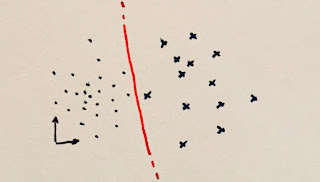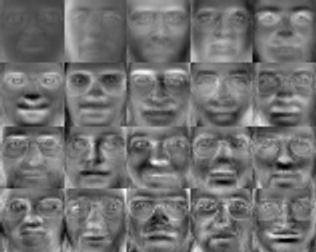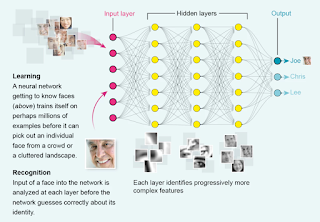## 26 March, 2017

### A programmer's sightseeing tour: Machine Learning and Deep Neural Networks (part 1)

TL;DR: You probably don't need DNNs, but you ABSOLUTELY SHOULD know and practice data analysis!

This won't be short...

- Machine Learning

Machine learning is a huge field nowadays, with lots of techniques and sub-disciplines. It would be very hard for me to provide an overview in a single article, and I certainly don't claim to know all about it.
The goal of this article is to introduce you to the basic concepts, just enough so we can orient ourselves and understand what we might need in our daily job as programmers.

I'll try to do so using terminology that is as much as possible close to what a programmer might expect instead of the grammar of machine learning which annoyingly often likes to call the same things in different ways based on the specific subdomain.
This is particularly a shame because as we'll soon see, lots of different fields, even disciplines that are not even usually considered to be "machine learning", are really intertwined and closely related.

- Supervised and unsupervised learning

The first thing we have to know is that there are two main kinds of machine learning: supervised and unsupervised learning.
Both deal with data, or if you wish, functions that we don't have direct access to but that we know through a number of samples of their outputs.

In the case of supervised learning, our data comes in the form of input->output pairs; each point is a vector of the unknown function inputs and it's labeled with the return value.
Our job is to learn a functional form that approximates the data; in other words, through data, we are learning a function that approximates a second unknown one.

Clearly supervised learning is closely related to function approximation. Another name for this is regression analysis or function fitting: we want to estimate the relationship between the input and output variables. Also related is (scattered) data interpolation and Kriging: in all cases we have some data points and we want to find a general function that underlies them.

Most of the times the actual methods that we use to fit functions to data come from numerical optimization: our model functions have a given number of degrees of freedom, flexibility to take different shapes, optimization is used to find the parameters that make the model as close as possible (minimize the error) to the data.Function fitting: 1D->1D
If the function's outputs are from a discrete set instead of being real numbers supervised learning is also called classification: our function takes an input and emits a class label (1, 2, 3,... or cats, dogs, squirrels,...), our job is, seen some examples of this classification at work, learn a way to do the same job on inputs that are outside the data set provided.Binary classifier: 2D->label
For unsupervised learning, on the other hand, the data is just made of points in space, we have no labels, no outputs, just a distribution of samples.

As we don't have outputs, fitting a function sounds harder, functions are relations of inputs to their outputs. What we could do though is to organize these points to discover relationships among themselves: maybe they form clusters, or maybe they span a given surface (manifold) in their n-dimensional space.

We can see clustering as a way of classifying data without knowing what the classes are, a-priori. We just notice that certain inputs are similar to each other, and we group these in a cluster.
Maybe later we can observe the points in the cluster and decide that it's made of cats, assign a label a-posteriori.2D Clustering
Closely related to clustering is dimensionality reduction (and dictionary learning/compressed sensing): if we have points in an n-dimensional space, and we can cluster them in k groups, where k is less than n, then probably we can express each point by saying how close to each group it is (projection), thus using k dimensions instead of n.2D->1D ProjectionEigenfaces
Dimensionality reduction is, in turn, closely related to finding manifolds: let's imagine that our data are points in three dimensions, but we observe that they all lie always on the unit sphere.
Without losing any information, we can express them as coordinates on the sphere surface (longitude and latitude), thus having saved one dimension by having noticed that our data lied on a parametric surface.

And (loosely speaking) all the times we can project points to a lower dimension we have in turn found a surface: if we take all the possible coordinates in the lower-dimensionality space they will map to some points of the higher-dimensionality one, generating a manifold.

Interestingly though unsupervised learning is also related to supervised learning in a way: if we think of our hidden, unknown function as a probability density one, and our data points as samples extracted according to said probability, then unsupervised learning really just wants to find an expression of that generating function. This is also the very definition of density estimation!

Finally, we could say that the two are also related to each other through the lens of dimensionality reduction, which can be seen as nothing else than a way to learn an identity function (inputs map to outputs) where we have the constraint that the function, internally, has to loose some information, has to have a bottleneck that ensures the input data is mapped to a small number of parameters.

- Function fitting

Confused yet? Head spinning? Don't worry. Now that we have seen that most of these fields are somewhat related, we can choose just one and look at some examples.

The idea that most programmers will be most familiar with is function fitting. We have some data, inputs and outputs, and we want to fit a function to it so that for any given input our function has the smallest possible error when compared with the outputs given.

This is commonly the realm of numerical optimization.

Let's say we suppose our data can be modeled as a line. A line has only two parameters: y=a*x+b, we want to find the values of a and b so that for each data point (x1,y1),(x2,y2)...(xN,yN), our error is minimized, for example, the L2 distance.
This is a very well studied problem, it's called linear regression, and in the way it's posed it's solvable using linear least squares.
Note: if instead of wanting to minimize the distance between the data output and the function output, we want to minimize the distance between the data points and the line itself, we end up with principal component analysis/singular value decomposition, a very important method for dimensionality reduction - again, all these fields are intertwined!

Now, you can imagine that if our data is very complicated, approximating it with a line won't really do much, we need more powerful models. Roughly speaking we can construct more powerful models in two ways: we either use more pieces of something simple, or we start using more complicated pieces.

So, on one extreme we can think of just using linear segments, but using many of them (fitting a piecewise linear curve), on the other hand, we can think instead of fitting higher-order polynomials, or rational function, or even to find an arbitrary function made of any combination of any number of operators (symbolic regression, often done via genetic programming).Polynomial versus piecewise linear.
The rule of the thumb is that simpler models have usually easier ways to fit (train), but might be wasteful and grow rather large (in terms of the number of parameters). More powerful models might be much harder to fit (global nonlinear optimization), but be more succinct.

- Neural Networks

For all the mystique there is around Neural Networks and their biological inspiration, the crux of the matter is that they are nothing more than a way to approximate functions, rather like many others, but made from a specific building block: the artificial neuron.

This neuron is conceptually very simple. At heart is a linear function: it takes a number of inputs, it multiplies them with a weight vector, it adds them together into a single number (a dot product!) and then it adds a bias value (optionally).
The only "twist" there is that after the linear part is done, a non-linear function (the activation function) is applied to the results.

If the activation function is a step (outputting one if the result was positive, zero otherwise), we have the simplest kind of neuron and the simplest neural classifier (a binary one, only two classes): the perceptron.Perceptron
In general, we can use many nonlinear functions as activations, depending on the task at hand.
Regardless of this choice though it should be clear that with a single neuron we can't do much, in fact, all we can ever do is express a distance from an hyperplane (again, we're doing a dot product), somewhat modified by the activation. The real power in neural networks come from the "network" part.Source
The idea is again simple: if we have N inputs, we can connect to them M neurons. These neurons will each give one output, so we end up with M outputs, and we can call this structure a neural "layer".
We can then rinse and repeat, the M outputs can be considered as inputs of a second layer of neurons and so on, till we decide enough is enough and at the final layer we use a number of outputs equal to the ones of the function we are seeking to approximate (often just one, but nothing prevents to learn vector-valued functions).

The first layer, connected to our input data, is unimaginatively called the input layer, the last one is called the output layer, and any layer in between is considered a "hidden" layer. Non-deep neural networks often employ a single hidden layer.

We could write down the entire neural network as a single formula, it would end up nothing more than a nested sequence of matrix multiplies and function applications. In this formula we'll have lots of unknowns, the weights we use in the matrix multiplies. The learning process is nothing else than optimization, we find the best weights that minimize the error of our neural network to the data given.

Because we typically have lots of weights, this is a rather large optimization problem, so typically fast, local, gradient-descent based optimizers are used. The idea is to start with an arbitrary set of weights and then update them by following the function partial derivatives towards a local minimum of the error.Source. See also this.
We need the partial derivatives for this process to work. It's impractical to compute them symbolically, so automatic differentiation is used, typically via a process called "backpropagation", but other methods could be used as well, or we can even have a mix of methods, using hand-written symbolic derivatives for certain parts where we know how to compute them, and automatic differentiation for other.

Under certain assumptions, it can be shown that a neural network with a single hidden layer is a universal approximator, it could (we might not be able to train it well, though...), with a finite (but potentially large number) of neurons approximate any continuous function on compact subsets of n-dimensional real spaces.

Part 2...